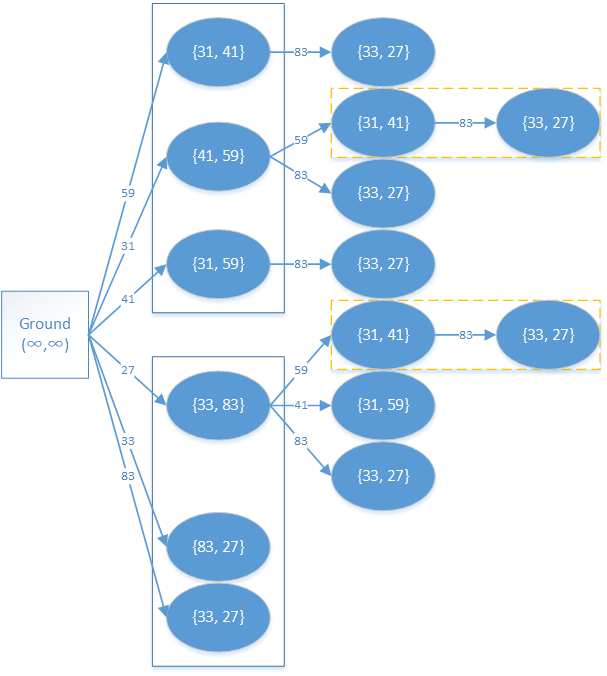# DAG 上的 DP

## 定义

DAG 即 有向无环图，一些实际问题中的二元关系都可使用 DAG 来建模，从而将这些问题转化为 DAG 上的最长（短）路问题。

## 解释种砖块，已知三条边长，每种都有无穷多个。要求选一些立方体摞成一根尽量高的柱子（每个砖块可以自行选择一条边作为高），使得每个砖块的底面长宽分别严格小于它下方砖块的底面长宽，求塔的最大高度。

## 过程

### 建立 DAG### 转移表示第块砖块在最上面，且采取第种堆叠方式时的最大高度。那么有如下转移方程：1 2 3 4 5 6 7 8 9 10 11 12 13 14 15 16 17 18 19 20 21 22 23 24 25 26 27 28 29 30 31 32 33 34 35 36 37 38 39 40 41 42 43 44 45 46 47 48 49 50 51 52 53 54 55 56 57 58 59 60 61 62 63 64 65 66 67 68 69 #include #include #include using namespace std; #define MAXN (30 + 5) #define MAXV (500 + 5) int d[MAXN]; int x[MAXN], y[MAXN], z[MAXN]; int babylon_sub(int c, int rot, int n) { if (d[c][rot] != -1) { return d[c][rot]; } d[c][rot] = 0; int base1, base2; if (rot == 0) { // 处理三个方向 base1 = x[c]; base2 = y[c]; } if (rot == 1) { base1 = y[c]; base2 = z[c]; } if (rot == 2) { base1 = x[c]; base2 = z[c]; } for (int i = 0; i < n; i++) { // 根据不同条件，分别调用不同的递归 if ((x[i] < base1 && y[i] < base2) || (y[i] < base1 && x[i] < base2)) d[c][rot] = max(d[c][rot], babylon_sub(i, 0, n) + z[i]); if ((y[i] < base1 && z[i] < base2) || (z[i] < base1 && y[i] < base2)) d[c][rot] = max(d[c][rot], babylon_sub(i, 1, n) + x[i]); if ((x[i] < base1 && z[i] < base2) || (z[i] < base1 && x[i] < base2)) d[c][rot] = max(d[c][rot], babylon_sub(i, 2, n) + y[i]); } return d[c][rot]; } int babylon(int n) { for (int i = 0; i < n; i++) { d[i] = -1; d[i] = -1; d[i] = -1; } int r = 0; for (int i = 0; i < n; i++) { // 三种建法 r = max(r, babylon_sub(i, 0, n) + z[i]); r = max(r, babylon_sub(i, 1, n) + x[i]); r = max(r, babylon_sub(i, 2, n) + y[i]); } return r; } int main() { int t = 0; while (true) { // 死循环求答案 int n; cin >> n; if (n == 0) break; // 没有砖头了就停止 t++; for (int i = 0; i < n; i++) { cin >> x[i] >> y[i] >> z[i]; } cout << "Case " << t << ":" << " maximum height = " << babylon(n); // 递归 cout << endl; } return 0; }